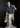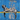# Stock Optimizer!February 19, 2020

AlgoDaily code challenge, Day 35.

#### Stock Buy and Sell Optimization

This is a classic technical interview question that I’ve seen a number of times in real life interviews. Let’s say you’re given an array of stock prices, with each element being an integer that represents a price in dollars.

``// [ 10, 7, 6, 2, 9, 4 ]``

Each index of the array represents a single day, and the the element at that index is the stock price for that given day. This means that the array below represents:

``````// const prices = [ 10, 7, 6, 2, 9, 4 ];
// Day 0 - a price of `\$10`
// Day 1 - `\$7`
// Day 2 - `\$6` (and so on...)``````

Given the ability to only buy once and sell once, our goal is to maximize the amount of profit (selling price - purchase price) that we can attain and return that value. Note the only caveat is that that we cannot sell a stock before we buy it, which is important in identifying a solution.

Can you write a stock optimizer method called `stockOptimizer`? See the following examples for further clarification:

``````// stockOptimizer([ 10, 7, 6, 2, 9, 4 ])
// 7``````

For the above example, the max profit is made when we buy on day/index `3`(when the price is `2`) and sell on day/index `4`(when the price is `9`) `9 - 2`gives us `7`, which is returned by the function. Let’s do another example.

``````stockOptimizer([9, 8, 6, 5, 3])
// 0``````

From a quick glance, it seems like there’s enough price differences to generate a profit. However, notice that the price drops every day, and thus we can’t have a profit since we’re required to buy before selling.

#### My Solution:

``````// Solution:

var stocksArray = [10, 7, 6, 4, 9, 4];
var highestPossibleProfit = 0;

function stockOptimizer(arr) {
for (i = 0; i < arr.length; i++) {
for (x = 1; x < arr.length; x++) {
if (
arr[i + x] - arr[i] > 0 &&
arr[i + x] - arr[i] > highestPossibleProfit
) {
highestPossibleProfit = arr[i + x] - arr[i];
}
}
}
}

stockOptimizer(stocksArray);

console.log("Stock Array = " + stocksArray);
console.log("Maximum possible profit = \$" + highestPossibleProfit);``````

Again, pretty simple. Use a nested for loop and keep a “highestPossibleProfit” integer variable. As the for loops run they check if the difference between the two current array elements is > than the current highestPossibleProfit integer.

Yep. Works, simple, clear. What’s not to love?

-mrWritten by Matthew Riddett who lives and works in Victoria BC, building fun and useful things. You can follow him on Twitter